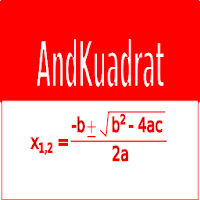AndKuadrat - Square Root Equation Calculator# AndKuadrat - Square Root Equation Calculator

## Calculating the roots of quadratic equation, theories and its explanations

App Size: 9.3M
Release Date: Jan 12, 2020
Price: Free
4.2
5 Ratings
Size
9.3M

Application for calculating the values ​​of the roots of quadratic equations, the determinants, types and properties of the roots of quadratic equations and their theories and explanations